### 三、函数的连续性

1．单变量函数的连续性

[函数在一点连续] 函数f(x)在点x0的连续性有下面几种定义方法：

|f(x)-f(x0)|<ε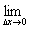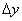=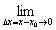[f(x0+Δx)(x0)]=0f(xn)=f(x0)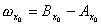=0

[函数在一点单边连续]f(x)=f(x0+0)=f(x0),则称f(x)在点x0右连续.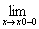f(x)=f(x00)=f(x0),则称f(x)在点x0左连续.

[函数在一个区间上连续]如果函数f(x)定义在区间[a,b]上，它在这个区间上任一点x都连续，那末称f(x)在区间[a,b]上连续（对开区间(a,b)可用同样定义）.

[函数的不连续（或间断）点] 由函数在一点连续的定义可知，间断点只能分两类：

[连续函数的运算]

[连续函数的性质]

4° 中间值定理 如果函数f(x)在闭区间[a,b]上连续，f(a)=Af(b)=B，并设KAB之间的任意一个值，那末在这个区间上至少存在一点x，使得f(x)的值等于K.特别是，若A,B不同号，则在这个区间内，至少有一点x,使得f(x)的值等于零.

5° 反函数的连续性 如果函数y=f(x)在区间[a,b]上递增而且连续，又f(a)=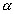f(b)=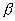，那末反函数x=(y)在区间[,]上也连续.

6° 以参数表出的函数的连续性 如果函数(t)和(t)在区间(,)内定义并连续，且函数(t)在此区间内是严格单调的，那末方程组

x=(t),y=(t)

y=[-1(x)]

[初等函数的连续性] 一般地说，基本初等函数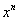,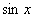,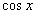,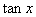,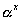,,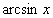,,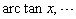以及由它们经过有限次的算术运算和复合函数运算后所得的函数，在一切使它们有意义的点都连续.

[实数集合的确界]

1° 有界集合 设有某一个实数集合E，若有一个数M，使得集合E的一切数，则称集合E是有上界的.类似地，若有一个数m,使得集合E的一切数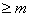，则称这个集合是有下界的.既有上界又有下界的集合称为有界集合.

2° 实数集合的上确界和下确界

E中没有小于的数，但是无论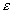>0多么小，E中总有小于+ε的数，则α称为集合E的下确界，记作infE.

[函数的一致连续性]

1° 函数的一致连续性定义 设函数f(x)定义在某一区间X(闭的或不闭的，有穷的或无穷的）上，若对任意给定的ε>0，都存在一个只与有关的δ=δ(ε)>0，使得对区间X上的任意两点x1x2，只要

|x2-x1|<δ

|f(x2)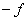(x1)|<ε

3°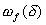=sup|f(x1)-f(x2)|